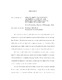## Strong shift equivalence, algebraic K-theory, and isolating zero-dimensional dynamics on manifoldsWe study the relations of shift equivalence and strong shift equivalence for matrices over a ring $\mathcal{R}$, and establish a connection between these relations and algebraic K-theory. We utilize this connection to obtain results in two areas where the shift and strong shift equivalence relations play an important role: the study of finite group extensions of shifts of finite type, and the Generalized Spectral Conjectures of Boyle and Handelman for nonnegative matrices over subrings of the real numbers. We show the refinement of the shift equivalence class of a matrix $A$ over a ring $\mathcal{R}$ by strong shift equivalence classes over the ring is classified by a quotient $NK_{1}(\mathcal{R}) / E(A,\mathcal{R})$ of the algebraic K-group $NK_{1}(\calR)$. We use the K-theory of non-commutative localizations to show that in certain cases the subgroup $E(A,\mathcal{R})$ must vanish, including the case $A$ is invertible over $\mathcal{R}$. We use the K-theory connection to clarify the structure of algebraic invariants for finite group extensions of shifts of finite type. In particular, we give a strong negative answer to a question of Parry, who asked whether the dynamical zeta function determines up to finitely many topological conjugacy classes the extensions by $G$ of a fixed mixing shift of finite type. We apply the K-theory connection to prove the equivalence of a strong and weak form of the Generalized Spectral Conjecture of Boyle and Handelman for primitive matrices over subrings of $\mathbb{R}$. We construct explicit matrices whose class in the algebraic K-group $NK_{1}(\mathcal{R})$ is non-zero for certain rings $\mathcal{R}$ motivated by applications. We study the possible dynamics of the restriction of a homeomorphism of a compact manifold to an isolated zero-dimensional set. We prove that for $n \ge 3$ every compact zero-dimensional system can arise as an isolated invariant set for a homeomorphism of a compact $n$-manifold. In dimension two, we provide obstructions and examples.